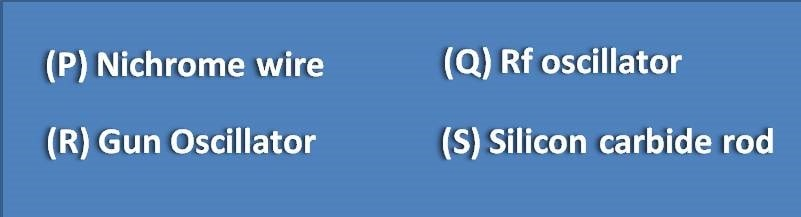# MCQ on ESR spectroscopy: Page-3

Source used in ESR to produce monochromatic microwaves is(A) Q and R

(B) P and S

(C) R only

(D) Q only

Gun oscillator emits microwaves which are used as source in ESR. Klystron tube is one type of gun oscillator. For NMR spectroscopy, Rf oscillator is used which produces radio waves.

Hyperfine splitting that can be observed with two equivalent protons will be

(A) 2

(B) 3

(C) 5

(D) 6

Number of hyperfine splitting can be given by formula 2nI + 1 where n is the number of equivalent nuclei and I is the spin quantum number of nuclei. So here, n=2 and I value for proton is ½ . So the number of peak splittings will be equal to 2(2)(1/2) + 1=3.

As operating frequency increases, hyperfine splitting constant

(A) Increases

(B) Decreases

(C) Remains constant

(D) Either increases or decreases

Primary standard used in ESR is

(A) 1,1-diphenyl-2’-picrylhydrazyl

(B) Nitrosobenzene

(C) Tetramethyl silane

(D) Diphenyl hydrazyl

The ratio of peak splitting that can observed with three equivalent protons will be

(A) 1:1

(B) 1:2:1

(C) 1:3:1

(D) 1:3:3:1